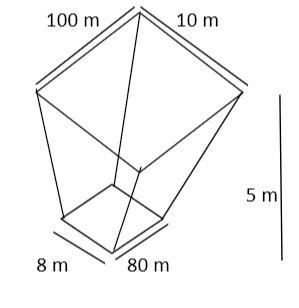QuestionAnswers

# A frustum of a pyramid has an upper base 100m by 10m and a lower base of 80m by 8m, if the altitude of the frustum is 5m, find the volume of the frustum (in cu. m.)A. 4567.67B. 3873.33C. 4066.67D. 2345.98Verified
128.4k+ views
Hint: In this particular problem apply the direct formula for the volume of frustum that is $Volume = \dfrac{h}{3}\left( {{A_1} + {A_2} + \sqrt {{A_1} \times {A_2}} } \right)$, where h is the altitude of the frustum, ${A_1}$ and ${A_2}$ are the areas of the upper and lower base of the frustum.As we know that to find the volume of the frustum first, we had to find the area of its upper and lower base.
Now as we can see from the above figure that the lower and upper base of the frustum is in rectangle shape.
And the area of the rectangle is calculated as $Area = Length \times Breadth$.
So, let the area of the upper base of the rectangle be ${A_1}$.
So, ${A_1} = 100 \times 10 = 1000{m^2}$
And let the area of the lower base of the rectangle be ${A_2}$.
So, ${A_2} = 80 \times 8 = 640{m^2}$
And it is given that the height (altitude) of the frustum is 5m.
So, now let us the put the values in the formula of volume of frustum that is $\dfrac{h}{3}\left( {{A_1} + {A_2} + \sqrt {{A_1} \times {A_2}} } \right)$
So, the volume of the frustum will be = $= \dfrac{5}{3}\left( {1000 + 640 + \sqrt {1000 \times 640} } \right) = \dfrac{5}{3}\left( {1640 + \sqrt {640000} } \right)$
$\Rightarrow$Volume = $= \dfrac{5}{3}\left( {1640 + 800} \right) = \dfrac{5}{3}\left( {2440} \right) = \dfrac{{12200}}{3} = 4066.66{m^3}$

Hence, the correct option will be C.

Note: Whenever we face such types of problems then first, we have to find the area of the rectangle using the formula $Area = Length \times Breadth$. And after that we can directly put the value of area and height in the formula to find the volume of the frustum and note that the formula for volume is the same for both cases (Lower base is more than upper base or upper base is more than lower base). This will be the easiest and efficient way to find the solution of the problem.